# How to Highlight Cell if Same Value Exists in Another Column in Excel

This post will guide you how to highlight cell if same value exists in another column in Excel. How do I highlight cell if value is present in any cell in another column in Excel 2013/2016.

## Highlight Cell If Same Value Exists in Another Column

Assuming that you have a list of data in range A1:B5, you want to highlight cell in Column A if the value is found in Column B, how to accomplish it. You can use the Conditional Formatting feature to highlight cell in Excel. Just do the following steps:

Step1: select your range of cells in which you wish to highlight.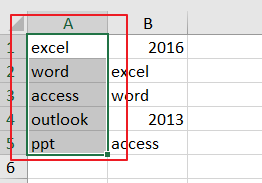Step2: go to Home tab, and click Conditional Formatting command under Styles group, and select new Rule from the context menu list. and the New Formatting Rule dialog will open.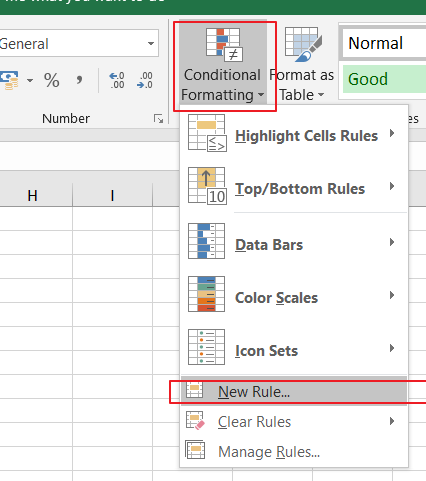Step3: click Use a formula to determine which cells to format option in the Select a Rule Type list, and type the following formula into the Format values where this formula is true text box.

=NOT(ISNA(VLOOKUP(A1,\$B:\$B,1,FALSE)))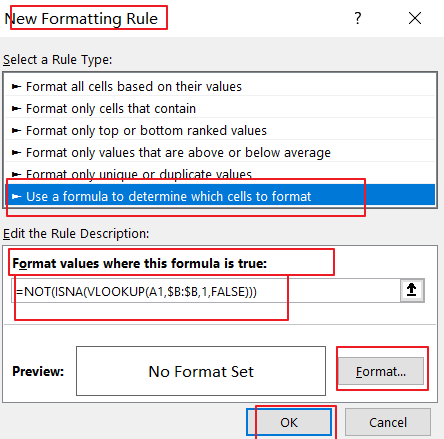Note: the Cell A1 is the first cell of the column that you want to highlight, and \$B:\$B is another column that you want to be checked)

Step4: click Format button in the New Formatting Rule dialog box, and the Format Cell dialog will open.

Step5: switch to Fill tab in the Format Cell dialog box, and choose one color as you need. click Ok button back to the New Formatting Rule dialog box.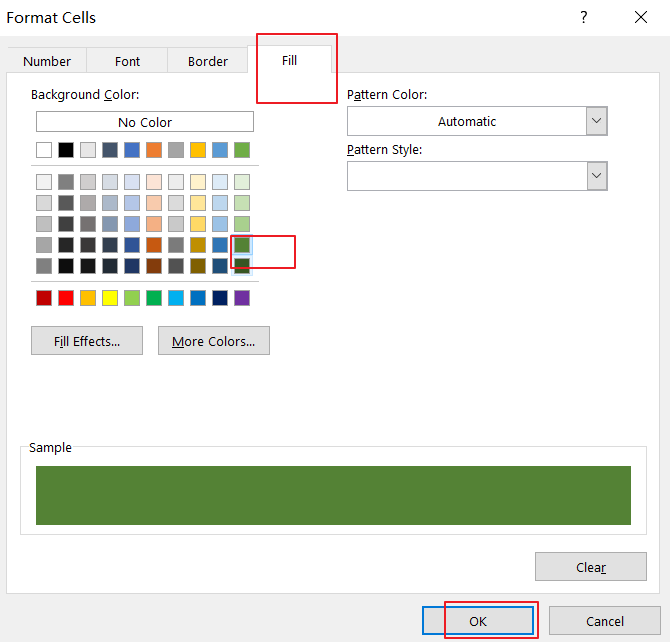Step6: click OK button. You would see that the cells in Column A have been highlighted if those values can be found in column B.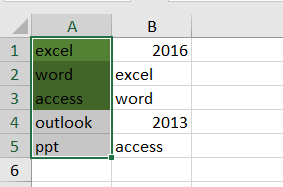## Highlight Cell If Same Value Exists in Another Column Using VBA

You can also use an Excel VBA Macro to highlight cell if value is present in another column. Just do the following steps:

Step1: open your excel workbook and then click on “Visual Basic” command under DEVELOPER Tab, or just press “ALT+F11” shortcut.Step2: then the “Visual Basic Editor” window will appear.

Step3: click “Insert” ->”Module” to create a new module.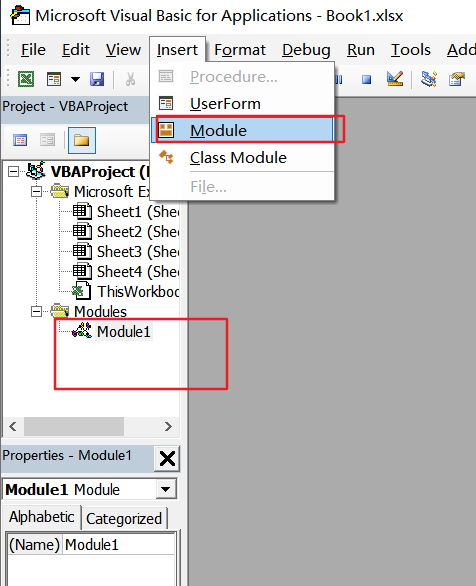Step4: paste the below VBA code  into the code window. Then clicking “Save” button.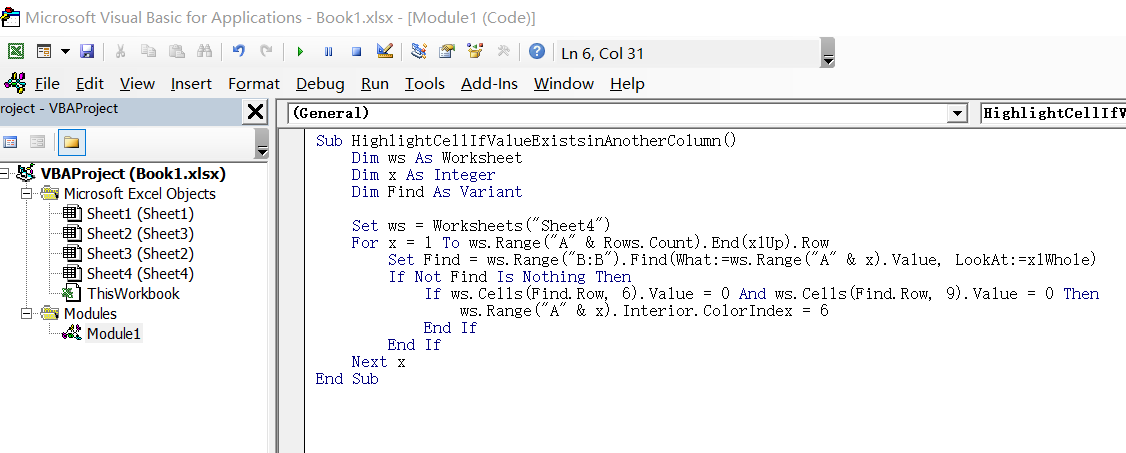```Sub HighlightCellIfValueExistsinAnotherColumn()
Dim ws As Worksheet
Dim x As Integer
Dim Find As Variant
Set ws = Worksheets("Sheet4")
For x = 1 To ws.Range("A" & Rows.Count).End(xlUp).Row
Set Find = ws.Range("B:B").Find(What:=ws.Range("A" & x).Value, LookAt:=xlWhole)
If Not Find Is Nothing Then
If ws.Cells(Find.Row, 6).Value = 0 And ws.Cells(Find.Row, 9).Value = 0 Then
ws.Range("A" & x).Interior.ColorIndex = 6
End If
End If
Next x
End Sub```

Step5: back to the current worksheet, then run the above excel macro. Click Run button.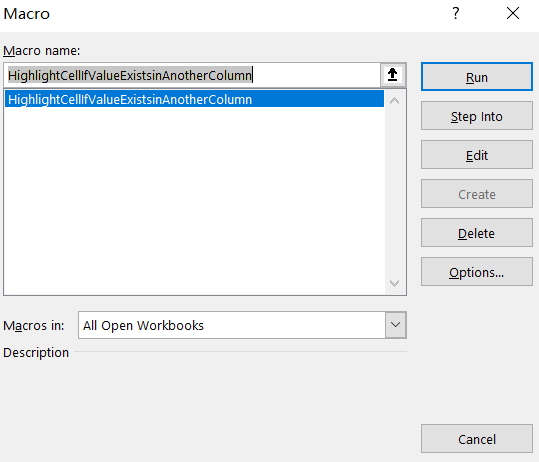Step6: let’s see the result: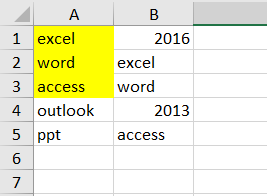### Related Functions

• Excel VLOOKUP function
The Excel VLOOKUP function lookup a value in the first column of the table and return the value in the same row based on index_num position.The syntax of the VLOOKUP function is as below:= VLOOKUP (lookup_value, table_array, column_index_num,[range_lookup])….
• Excel ISNA function
The Excel ISNA function used to check if a cell contains the #N/A error, if so, returns TRUE; otherwise, the ISNA function returns FALSE.The syntax of the ISNA function is as below:=ISNA(value)….